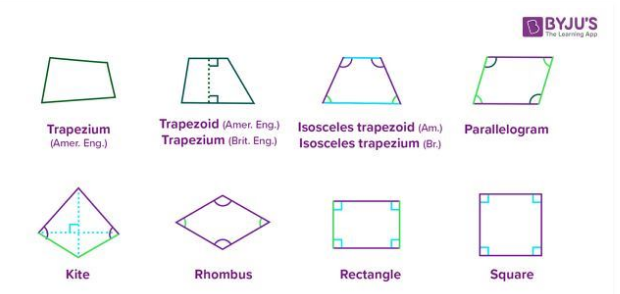Quadrilaterals are a two-dimensional plane figure, which has four edges and four vertices. The sides of the quadrilaterals are straight lines and joint with each other end to end. The word ‘quad’ states as four. It is nothing but a polygon which has four corners and four sides. These quadrilaterals can be seen in different shapes, regular or irregular. The examples are a kite, a deck of cards, etc. We will learn the types of quadrilaterals here.

• It has four sides, four corners/vertices and four angles
• It has both regular and irregular shape
• Total of all its interior angles is equal to 360 degrees

There are various types of quadrilaterals, such as:

1. Trapezium: One of the opposite sides are parallel
2. Parallelogram: Both the opposite sides are parallel and equal in length.
3. Squares: All the sides are equal and the angles are at 90 degrees.
4. Rectangle: Opposite sides are equal and the angles are at 90 degrees.
5. Rhombus: All the sides are equal and diagonals bisect at 90 degrees.
6. Kite: Two pairs of adjacent/neighbouring sides are of equal length.Area of Quadrilateral: The region occupied by any type of the quadrilateral in a two-dimensional plane is its area. The formulas for different types of quadrilaterals are given by:

 Area of a Parallelogram Base x Height Area of a Rectangle Length x Width Area of a Square Side x Side Area of a Rhombus 1/2 x Diagonal 1 x Diagonal 2 OR Base x Height Area of a Kite 1/2 x Diagonal 1 x Diagonal 2

Q.1: Find the area of the rectangle whose length is 4 cm and width is 3.5 cm.

Solution: Given, Length of the rectangle = 4 cm

And width of the rectangle = 3.5 cm

Since, area of the rectangle, A = Length x Width

A = 4 cm x 3.5 cm

A = 14 cm2

Q.2 If the area of the square is 121 cm2, then find the length of its sides.

Solution: Given, Area of the square = 121 cm2

We know,

Area of the square = side2

Therefore,

121 = side2

Or Side = √121 = 11

Hence, the side of the square is 11 cm.